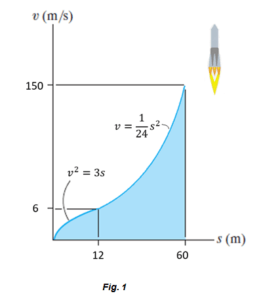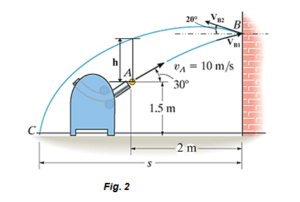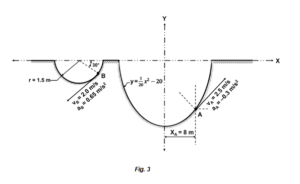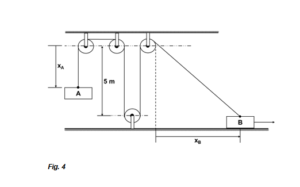Avail 15% off on First assignment order NAH_FIRST_15%

 University University Of Canterbury (UC) Subject Mechanical Principles B - Dynamics

Question 1

The Rocket travels along a straight line with the velocity changing with displacement as depicted by the graph shown in Fig. 1. Construct the st and as graphs. Also, determine the time required for the rocket to travel a distance s = 60 m if s0= 0 when t = 0.Question 2

The pitching machine throws the 0.5 kg ball towards the wall with an initial velocity VA= 10 m/s at 300 to the horizontal as shown. Calculate the velocity VB1with which the ball will hit the wall at B. After striking the wall at B, the ball deflects backward at a velocity of VB2 = 5.0 m/s at an angle of 200 to the horizontal and follows the projectile path shown from B to C. Calculate the height h at which the ball is above point A in the return flight and the distance S at which it will hit the ground from the wall.### Stuck! Do not Know Assessment Answers?

Question 3

Two particles A and B are moving along two curvilinear paths as shown in Fig 3.  At the given instant, the tangential velocity and acceleration of the particle at A are 2.5 m/s and -0.3 m/s2 respectively, and act upwards, as shown. Point A is at XA = 8.0 m. At the same instant, particle B moving along the semi-circular path has velocity and acceleration equal to 2.0 m/s and 0.65 m/s2, acting downwards, as shown in Fig. 3. Calculate the relative acceleration of particle B with respect to that of particle A at the instant given.Question 4

Mass A is raised by moving the mass B towards the right, based on the rope and pulley arrangement shown in Fig. 4. When XB =0, both blocks A and B will rest on the bottom surface with the free lengths of the rope on either side equal to 5 m as shown. The velocity of B varies as the function:

Calculate the velocity of mass A when the time t = 0.90 seconds. Neglect friction and masses of the rope and the pulleys.### Buy Custom Assignment & Homework Solutions

Pay to NZ Native Writers | Cheap Cost & Plag Free

Get Help By Expert

Get instant assignment help on Mechanical Principles B - Dynamic in New Zealand. Our Canterbury assignment writers serve you the best quality and error-free assignment help. They will assist you 24 hours a day and 7 days a week to get an A+ grade in your education field.